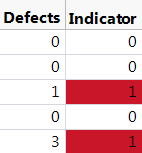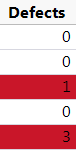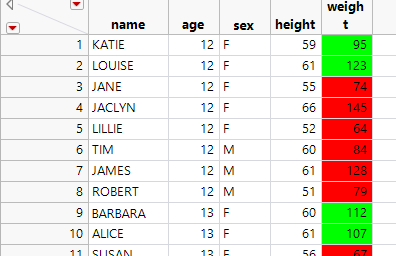Our World Statistics Day conversations have been a great reminder of how much statistics can inform our lives. Do you have an example of how statistics has made a difference in your life? Share your story with the Community!
Choose Language Hide Translation Bar
Highlighted

## Color Cells based on formula result

Hi everyone,

I have a column (lets call it Indicator) with a formula in it that returns a 0 or a 1.

Right now I know how to do this:

`formulaResult = :Indicator << Get Values;:Indicator << Color Cells( "red", Loc(formulaResult==1) );`

But what I really want to do is have the data table dynamically respond: if I change the data so that the Indicator switches to 0 (false), I don't want the cell to highlight any more, and if I add more data to the table and the formula calculates, I want the 1's to highlight.

I can't figure out how to do this with Color Cells. I thought that perhaps a strange implementation of the Color Gradient column property might work but after playing around with that for a while I couldn't get the result I wanted. Looking for some ideas.

Michael

2 ACCEPTED SOLUTIONS

Accepted Solutions
Highlighted

## Re: Color Cells based on formula result

So basically I'm just faking a color gradient that gradients over a tiny window.  So for only two colors you only need 4 points. 0, some number very close to 0, some number very close to 1, and 1.  If you wanted more than 2 colors you'd have to calculate values from 0 to 1 at each point in your range you wanted.  for instance if you wanted 110, it you'd have to add two values at 10/35 and 10/35+.0000001

``````Names Default to Here(1);
dt = Open("\$SAMPLE_DATA\Big Class.jmp");
Column(dt, "weight") << Set Property(
{{"Something", 16387,
{{255, 0, 0}, {0, 255, 0}, {0, 255,	0}, {255, 0, 0}}, //list of colors
{0, .00000001, .9999999, 1}}, //corresponding locations from 0 to 1
Range( {100, 135, 117.5} ) //minimum, maximum and center.  0, 1, and .5 is another way to look at it

}
);
Column(dt, "weight") << Color Cell By Value(1);``````

Vince Faller - Predictum
Highlighted

## Re: Color Cells based on formula result

Here's the same approach with a white-coloring Else clause. The hidden column is here numeric by default so both the Then and the Else must return something numeric, e.g. a missing value.

``````dt = Open("\$SAMPLE_DATA\Big Class.jmp");
dt << New Column("highlight cells",
Formula(If(100 < :weight < 135, :weight << color cells("Red", Row()); .,
:weight << color cells("White", Row()); .)),
Hide(1)
);``````

17 REPLIES 17
Highlighted

## Re: Color Cells based on formula result

Try the "Value Colors" property with "Color Cells by Value" checked.

Example:

``````// Example table
dt = New Table("test",
New Column("data", Formula(Random Uniform())),
New Column("Indicator",
Set Property("Value Colors", {. = -12632256}),
Formula(:data < 0.5),
Color Cell by Value
)
);

// Test if dynamic
Wait(1);
:data << eval formula;
Wait(1);

Highlighted

## Re: Color Cells based on formula result

Awesome! I think I oversimplified my problem a bit. Let's try this generalization.

Now my Indicator is now a count of defects (0, 1, 2, 3, 4, etc.). How can I color everything >=1 with the same color, and not color the zeroes?

Highlighted

## Re: Color Cells based on formula result

You just need to change the logic used in the column formula, for example, :data > 0.
Learn it once, use it forever!
Highlighted

## Re: Color Cells based on formula result

Mark,

Of course. It makes sense that I could just create another indicator column. The only problem is this will color the cells in the indicator column rather than highlighting the number of defects, like this:What I'd prefer is this:Any ideas?

Highlighted

## Re: Color Cells based on formula result

Make a list that defines the value colors the way you need, e.g. white for 0 and red for integers > 0. The Value Colors property seems to require that each value to be colored has its own "assignment". Thus, all possible values of "Defects" must be included in the list.

Example:

``````// Make list that defines the Value Colors
n = 10; //set highest conceivable nr of defects
vc = {0 = 2}; // White cell color for no defects
For(i = 1, i <= n, i++,
Insert Into(vc, Eval Expr(Expr(i) = 19)) // red for defects 0
);

// Example table
dt = New Table("test",
New Column("Defects", Formula(Random Integer(0, 3)), Set Property("Value Colors", vc), Color Cell by Value)
);
``````

Highlighted

## Re: Color Cells based on formula result

For counting defects I can work with this. Sometimes I also have continuous variables that I need to work with and this solution won't work there but maybe the color gradient would work okay in that situation.

Highlighted

## Re: Color Cells based on formula result

This issue is coming up again already for me just a week later.

The new application is that we have a number of situations where we want to flag values that are out of spec. Neither color gradient nor value colors do a great job of this. We can certainly create a new column and color based on 0 vs 1, but if I have multiple variables with specs to enforce, I am going to have to create a new column for each variable. This will become unwieldy quickly! I'd like a better solution.

Highlighted

## Re: Color Cells based on formula result

``````Names Default to Here(1);
dt = Open("\$SAMPLE_DATA\Big Class.jmp");
Column(dt, "weight") << Set Property(
{{"Something", 16387, {{255, 0, 0}, {255, 0, 0}, {0, 255, 0}, {0, 255,
0}, {255, 0, 0}, {255, 0, 0}}, {0, 0.33333333, 0.33333334,
0.666666666666, 0.666666666667, 1}}, Range( {64, 172, 105} )

}
);
Column(dt, "weight") << Color Cell By Value(1);``````Vince Faller - Predictum
Highlighted

## Re: Color Cells based on formula result

This is closer. It appears that the green range is approx. 92-127 for this example. Suppose my spec range is 100-135. How do I do the math to figure out the endpoints to feed into the Range function in Color Gradient? (And then I have the pain point of having to explain to other JMP users here why they need to turn two numbers, i.e., 100 and 135, into three values to satisfy the Range function.)

Article Labels

There are no labels assigned to this post.